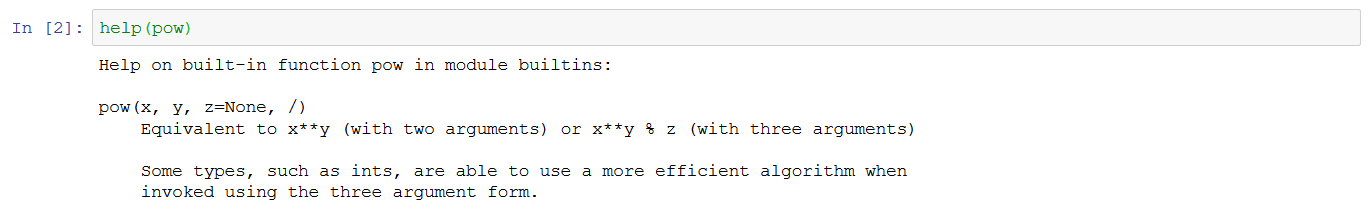Mis à jour le 18/02/2022

## Understand parameters and return values

Connectez-vous ou inscrivez-vous gratuitement pour bénéficier de toutes les fonctionnalités de ce cours !Since the beginning of this course, we have been using functions, such as `print()` or  `len()`. In this chapter, you will learn the different components of a method declaration (parameters and return value) and the difference between a value type and a reference type.

Let's say you have a 6 (length) by 4 (width) rectangle and you want to print its perimeter. You can create a function which calculates it! Let's see how it's done in Python:

``````def displayPerimeter():
length = 6
width = 4
perimeter = 2*(length + width)
print(perimeter) # -> 6}
``````

This function is implemented correctly, but quite useless as you'll always end up working with the same numbers.

#### Functions with parameters

To address this limitation, you need to make your function accept outside numbers. You can do that by defining a function with parameters.

In Python, the variable name and type of the parameter are part of function declaration. Here's what it looks like:

``````def displayPerimeter(length, width):
perimeter = 2 * (length + width)
print(perimeter) # -> depends on the values of length and width}
``````

Parameters are variables listed in the function declaration that are specified inside  `()`  by the name. Now you can call this function and pass to it any values you want:

``````displayPerimeter(10, 11) # -> 42
displayPerimeter(2, 2) # -> 8
``````

Each value is assigned to a parameter in the order they are defined.

Parameters are the variables declared in a function. And the values that are passed to that function are called arguments.

Now, what can you do about the result?

Often, the code that called the function needs an answer to perform its own work. This answer can be provided by the return value of the function.

#### Define a return value

To define a return value, you need to:

1. Alter the declaration of the function to indicate that it's expected to return a result.

2. End the function with the return keyword.

Here is how you can turn your `displayPerimeter` into a `calculatePerimeter` function that will return the result of the calculation so it can be used by the calling function:

``````def sumCustom(a, b):
calc = a + b
return calc
``````

You could also forgo the sum variable and return the result of the calculation expression directly:

``````def sumCustom(a, b):
return a + b
``````

Once your function is defined, you can use it as many times as you want. Here are a couple of variations:

``````sumOfSmallNumbers = sumCustom(3,7)
print(sumOfSmallNumbers) # -> 10

sumOfLargerNumbers = sumCustom(sumOfSmallNumbers,999)
print(sumOfLargerNumbers) # -> 1009
``````

In the example above, we used the result of the first calculation as a parameter for the next one.

You already know `print``len`, and `input`, which are standard Python functions. There are way more functions, like:

• `sum` : calculates the sum of a list

• `pow` : takes two arguments, x a number, and y a number which is to be powered with x. It's equivalent to `x**y`

• `abs` : returns the absolute value of a given number

For instance:

``````myList = [1,2,3,4,-2]

sum(myList) # -> 8
pow(2,3) # -> 8, equivalent to 2**3
abs(-3) # -> 3
``````

You may remember the name of a function, but not always what it is supposed to do. Don't panic! The `help` function is there to... help! If you call `help(myFunctionName)`, it will display the help documentation about this function:

• Its purpose

• Use recommendations

• List and parameter descriptions

• And more

Here is an example with the `pow` function:There is a lot of new knowledge here; you are progressing so fast!

In this chapter, you learned:

• Functions can have parameters and return values.

• A return value is a result of the function's execution. It can be returned to the block of code that called the function, and then used as needed.

• Parameters are the necessary input for a function to be executed and produce a result.

• Parameters are variables defined by name. They are specified in the function's declaration.

• When calling a function, you are passing values to those variables. Those values are called arguments.

• You can use the help function to display the function's documentation.